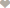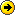# Nigeria Student Forum

Welcome, Guest: Join Nigeria Student Forum / Login / Trending Now / Recent

Stats: 17,629 members, 70,753 Topics, 15,563 Comments. Date: Jul 17 2019, 3:46 am

## Jamb Mathematics Syllabus For 2018/2019 Jamb Examination

Nigeria Student Forum / Academic Tips / Jamb Updates / Jamb Mathematics Syllabus For 2018/2019 Jamb Examination

(Go Down)
Fadeyi OluwaseunB.tech Marketing Management
200Level, Nigeria.

The Jamb Mathematics Syllabus for 2018/2019 Jamb examination has been released. Checkout topics to read to prepare for 2018/2019 JAMB exam. The aim of the Unified Tertiary Matriculation Examination (UTME) syllabus in Mathematics is to prepare the candidates for the Board’s examination.
It is designed to test the achievement of the course objectives, which are to:
(1) acquire computational and manipulative skills;
(2) develop precise, logical and formal reasoning skills;
(3) apply mathematical concepts to resolve issues in daily living;

This syllabus is divided into five sections:

I. Number and Numeration.
II. Algebra
III. Geometry/Trigonometry.
IV. Calculus
V. Statistics

TOPICS/CONTENTS/NOTES OBJECTIVES CURRENT MATHEMATICS SYLLABUS FOR JAMB 2018/2019

SECTION I: NUMBER AND NUMERATION
1. Number bases:
(a) operations in different number bases from 2 to 10;
(b) conversion from one base to another including fractional parts.
Candidates should be able to:
i. perform four basic operations (x,+,-,÷)
ii. convert one base to another.
2. Fractions, Decimals, Approximations and Percentages:
(a) fractions and decimals;
(b) significant figures;
(c) decimal places;
(d) percentage errors;
(e) simple interest;
(f) profit and loss percent;
(g) ratio, proportion and rate;
(h) shares and valued added tax (VAT).
Candidates should be able to:
i. perform basic operations
(x,+,-,÷) on fractions and decimals;
ii. express to specified number of significant figures and decimal places;
iii. calculate simple interest, profit and loss percent; ratio proportion and rate;
iv. Solve problems involving share and VAT.
3. Indices, Logarithms and Surds:
(a) laws of indices;
(b) standard form;
(c) laws of logarithm;
(d) logarithm of any positive number to a given base;
(e) change of bases in logarithm and application;
(f) relationship between indices and logarithm;
(g) surds.
Candidates should be able to:
i. apply the laws of indices in calculation;
ii. establish the relationship between indices and logarithms in solving problems;
iii. solve problems in different bases in logarithms;
iv. simplify and rationalize surds;
v. perform basic operations on surds.
4. Sets:
(a) types of sets
(b) algebra of sets
(c) venn diagrams and their applications.
Candidates should be able to:
i. identify types of sets, i.e empty, universal, complements, subsets, finite, infinite and disjoint sets;
ii. solve problems involving cardinality of sets;
iii. solve set problems using symbol;
iv. use venn diagrams to solve problems involving not more than 3 sets.

1. Polynomials:
(a) change of subject of formula
(b) factor and remainder theorems
(c) factorization of polynomials of degree not exceeding 3.
(d) multiplication and division of polynomials
(e) roots of polynomials not exceeding degree 3
(f) simultaneous equations including one linear one quadratic;
(g) graphs of polynomials of degree not greater than 3.
Candidates should be able to:
i. find the subject of the formula of a given equation;
ii. apply factor and remainder theorem to factorize a given expression;
iii. multiply and divide polynomials of degree not more than 3;
iv. factorize by regrouping difference of two squares, perfect squares and cubic expressions; etc.
v. solve simultaneous equations – one linear, one quadratic;
vi. interpret graphs of polynomials including applications to maximum and minimum values.

2. Variation:
(a) direct
(b) inverse
(c) joint
(d) partial
(e) percentage increase and decrease.
Candidates should be able to:
i. solve problems involving direct, inverse, joint and partial variations;
ii. solve problems on percentage increase and decrease in variation.
3. Inequalities:
(a) analytical and graphical solutions of linear inequalities;
(b) quadratic inequalities with integral roots only.
Candidates should be able to:
i. solve problems on linear and quadratic
inequalities;
ii. interprete graphs of inequalities.

4. Progression:
(a) nth term of a progression
(b) sum of A. P. and G. P.
Candidates should be able to:
i. determine the nth term of a progression;
ii. compute the sum of A. P. and G.P;
iii. sum to infinity of a given G.P.
5. Binary Operations:
(a) properties of closure, commutativity, associativity and distributivity;
(b) identity and inverse elements (simple cases only).
Candidates should be able to:
i. solve problems involving closure, commutativity, associativity and distributivity;
ii. solve problems involving identity and inverse elements.
6. Matrices and Determinants:
(a) algebra of matrices not exceeding 3 x 3;
(b) determinants of matrices not exceeding 3 x 3;
(c) inverses of 2 x 2 matrices
[excluding quadratic and higher degree equations].
Candidates should be able to:
i. perform basic operations (x,+,-,÷) on matrices;
ii. calculate determinants;
iii. compute inverses of 2 x 2 matrices.

SECTION III: GEOMETRY AND TRIGONOMETRY
1. Euclidean Geometry:
(a) Properties of angles and lines
(b) Polygons: triangles, quadrilaterals and general polygons;
(c) Circles: angle properties, cyclic quadrilaterals and intersecting chords;
(d) construction.
Candidates should be able to:
i. identify various types of lines and angles;
ii. solve problems involving polygons;
iii. calculate angles using circle theorems;
iv. identify construction procedures of special angles, e.g. 30°, 45°, 60°, 75°, 90° etc.

2. Mensuration:
(a) lengths and areas of plane geometrical figures;
(b) lengths of arcs and chords of a circle;
(c) Perimeters and areas of sectors and segments of circles;
(d) surface areas and volumes of simple solids and composite figures;
(e) the earth as a sphere:- longitudes and latitudes.
Candidates should be able to:
i. calculate the perimeters and areas of triangles, quadrilaterals, circles and composite figures;
ii. find the length of an arc, a chord, perimeters and areas of sectors and segments of circles;
iii. calculate total surface areas and volumes of cuboids, cylinders. cones, pyramids, prisms, spheres and composite figures;
iv. determine the distance between two points on the earth’s surface.
3. Loci:
locus in 2 dimensions based on geometric
principles relating to lines and curves.
Candidates should be able to:
identify and interpret loci relating to parallel lines, perpendicular bisectors, angle bisectors and circles.
4. Coordinate Geometry:
(a) midpoint and gradient of a line segment;
(b) distance between two points;
(c) parallel and perpendicular lines;
(d) equations of straight lines.
Candidates should be able to:
i. determine the midpoint and gradient of a line segment;
ii. find the distance between two points;
iii. identify conditions for parallelism and perpendicularity;
iv. find the equation of a line in the two-point form, point-slope form, slope intercept form and the general form.
5.Trigonometry:
(a) trigonometrical ratios of angels;
(b) angles of elevation and depression;
(c) bearings;
(d) areas and solutions of triangle;
(e) graphs of sine and cosine;
(f) sine and cosine formulae.
Candidates should be able to:
i. calculate the sine, cosine and tangent of angles between – 360° ≤ θθ ≤ 360°;
ii. apply these special angles, e.g. 30°, 45°, 60°, 75°, 90°, 105°, 135° to solve simple problems in trigonometry;
iii. solve problems involving angles of elevation and depression;
iv. solve problems involving bearings;
v. apply trigonometric formulae to find areas of triangles;
vi. solve problems involving sine and cosine graphs.

SECTION IV: CALCULUS
I. Differentiation:
(a) limit of a function
(b) differentiation of explicit
algebraic and simple
trigonometrical functions –
sine, cosine and tangent.
Candidates should be able to:
i. find the limit of a function
ii. differentiate explicit algebraic and simple trigonometrical functions.
2. Application of differentiation:
(a) rate of change;
(b) maxima and minima.
Candidates should be able to:
solve problems involving applications of rate of change, maxima and minima.
3. Integration:
(a) integration of explicit
algebraic and simple
trigonometrical functions;
(b) area under the curve.
Candidates should be able to:
i. solve problems of integration involving algebraic and simple trigonometric functions;
ii. calculate area under the curve (simple cases only).

SECTION V: STATISTICS
1. Representation of data:
(a) frequency distribution;
(b) histogram, bar chart and pie chart.
Candidates should be able to:
i. identify and interpret frequency distribution tables;
ii. interpret information on histogram, bar chat and pie chart
2. Measures of Location:
(a) mean, mode and median of ungrouped and grouped data – (simple cases only);
(b) cumulative frequency.
Candidates should be able to:
i. calculate the mean, mode and median of ungrouped and grouped data (simple cases only);
ii. use ogive to find the median, quartiles and percentiles.
3. Measures of Dispersion:
range, mean deviation, variance and standard deviation.
Candidates should be able to:
calculate the range, mean deviation, variance and standard deviation of ungrouped and grouped data.
4. Permutation and Combination:
(a) Linear and circular arrangements;
(b) Arrangements involving repeated objects.
Candidates should be able to:
solve simple problems involving permutation and combination.
5. Probability:
(a) experimental probability (tossing of coin,
throwing of a dice etc);
(b) Addition and multiplication of probabilities
(mutual and independent cases).
Candidates should be able to:
solve simple problems in probability (including addition and multiplication).

Recommended Text Books To Cover 2018/2019 Jamb Mathematics Syllabus.
Adelodun A. A (2000) Distinction in Mathematics: Comprehensive Revision Text, (3rd Edition) Ado -Ekiti: FNPL.
Anyebe, J. A. B (1998) Basic Mathematics for Senior Secondary Schools and Remedial Students in Higher/ institutions, Lagos: Kenny Moore.
Channon, J. B. Smith, A. M (2001) New General Mathematics for West Africa SSS 1 to 3, Lagos: Longman.
David -Osuagwu, M. et al (2000) New School Mathematics for Senior Secondary Schools, Onitsha: Africana – FIRST Publishers.
Egbe. E et al (2000) Further Mathematics, Onitsha: Africana – FIRST Publishers
Ibude, S. O. et al (2003) Agebra and Calculus for Schools and Colleges: LINCEL Publishers.
Tuttuh – Adegun M. R. et al (1997), Further Mathematics Project Books 1 to 3, Ibadan: NPS Educational

Source: https://ngstudentforum.com.ng/p/4950 LikeNgstudentforum.com Is The Next Big Hits Student Forum In Nigeria

E.g Seuncoded